# Primary 6 CA1 Practice #4

Time elapsed:
Q1. Express 17 as a percentage.     [1 mark]
Q2. AB is perpendicular to BC. Find ∠m     [1 mark]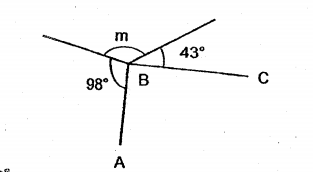Q3. The figure is made up of an equilateral triangle, a parallelogram and a triangle. Line AB is a straight line. Find the sum of ∠w, ∠x, ∠y and ∠z.     [2 marks]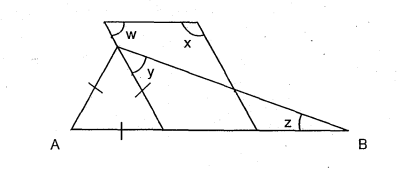Q4. At a cinema, the number of adults to the number of children was 2 : 5. Of the adults, the ratio of the number of men to the number of women was 2 : 3. What was the ratio of the number of men to the number of children?     [1 mark]
Q5. In a class of 40 pupils, 30 of them were girls. What percentage of the class were girls?     [1 mark]
Q6. Look at the pattern below. Find the sum of the two missing numbers.     [2 marks]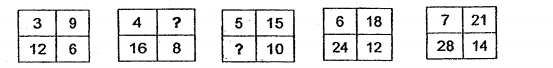Q7. A coffee machine can process 15 kg of coffee beans every minute. How many minutes will it take for the coffee machine to process 20 kg of coffee beans?     [1 mark]
Q8. Jasmine bought some flowers for her company event. 45% of the flowers are roses, 15% of the flowers are lily and the remaining flowers are sunflowers. Given that she bought 300 stalks of flowers in total, how many stalks of sunflowers did she buy?     [2 marks]
Q9. A tank was filled with 45 ℓ of water at 07 00. Water flowed out from the tank from 07 00 to 08 00 and from 09 00 to 11 00. The table below shows the volume of water in the tank from 07 00 to 11 00. Which one of the graphs below best represents the information in the table?     [2 marks]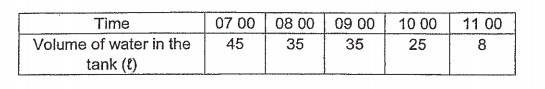Q10. Which lines represent the correct pair of height and base for the triangle ABD?     [1 mark]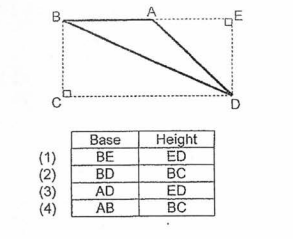Q11. There are 90 girls in the chess club. The number of girls is 20% more than the number of boys. How many pupils are there in the chess club?     [2 marks]
Q12. In a PE store, the number of basketballs was 4 times the number of soccer balls. When 14 of the basketballs went missing, there were still 20 more basketballs than soccer balls. How many basketball were left?     [1 mark]
Q13. In the number line below, what is the value of X as indicated by the arrow?     [1 mark]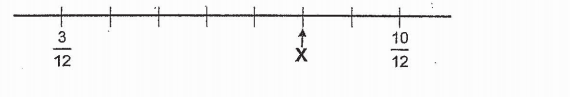Q14. Study the diagram below. Which button must be removed so that 50% of the remaining round buttons are white?     [1 mark]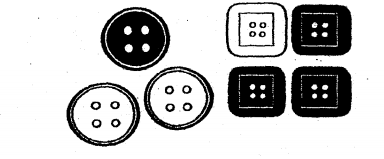Q15. There are 48 girls in the canteen. 25% of the children in the canteen are girls. How many boys are there?     [2 marks]
Q16. Yvette uses syrup and water in the ratio 3 : 5 to make lemon juice. She uses 330 ㎖ of syrup. How much water does she use?     [1 mark]
Q17. Look at the number line below. What is the value of X?     [1 mark]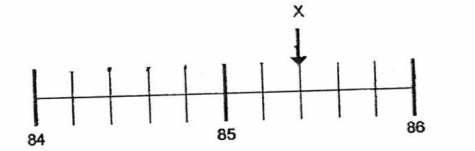Q18. Find the value of 8 + p/2 when p = 4.     [1 mark]
Q19. Find the value of 140 x 4000.     [1 mark]
Q20. The ratio of the number of pens to pencils to rulers in a stationery shop is 5 : 6 : 3. There are 240 more pencils than rulers. How many pens are there in the shop?     [2 marks]